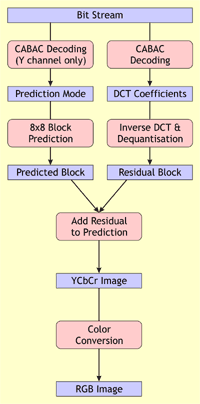# AIC - Algorithm Details

These pages describe the algorithms used by the Advanced Image Coding codec. Note that these algorithms are not new to this codec. Existing algorithms from the H.264 and JPEG standards are combined in the AIC codec, although usually modified a bit to fit the needs of the codec.

The following figures show the process flow of the AIC encoder and decoder:AIC Encoder AIC Decoder

Both encoder and decoder share the same functional elements:

• Color Conversion.
A source RGB image is transformed to the YCbCr color space that has less entropy in the chominance channels (Cb and Cr) and thus makes these channels better compressible. This only applies to color images. Grayscale images have only 1 channel.
• Block Prediction.
Every channel (Y, Cb and Cr for color images, or Y for grayscale images) is split into blocks of 8x8 pixels. The contents of each block is predicted from previously encoded and decoded blocks in the channel. There are 9 ways to predict the current block. The prediction mode that minimizes the differences between the original and predicted block is chosen. To save bits on coding the prediction mode, the blocks in the Cb and Cr channels use the same prediction modes as the corresponding blocks in the Y channel.
The values in the predicted block are subtracted from the ones in the original block to form a residual block. When a good prediction mode is chosen, the values in this residual block are smaller than the original pixel values and thus better compressible.
• Discrete Cosine Transform (DCT) and Quantisation.
To further reduce the entropy of the residual block, this block is transformed using the Discrete Cosine Transform. The resulting DCT coefficients are usually smaller than the original values. By quantising these coefficients with a certain value based on a chosen quality level, the coefficients are reduced even further. As a result, many of the coefficients in the block will be zero, and most others will be close to zero.
• Context Adaptive Binary Arithmetic Coding (CABAC).
The prediction mode and quantised DCT coefficients are finally encoded to the stream using a binary arithmetic coding scheme that adapts to the context in which these values are coded. This means that commonly used prediction modes and DCT coefficients are coded using fewer bits than rare prediction modes and coefficients.